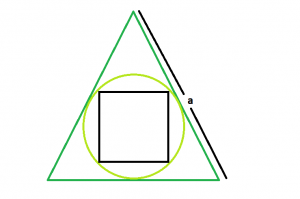GFG App
Open AppBrowser
Continue

# Area of a square inscribed in a circle which is inscribed in an equilateral triangle

Given here is an equilateral triangle with side length a, which inscribes a circle, which in turn inscribes a square. The task is to find the area of this square.
Examples:

```Input: a = 6
Output: 1

Input: a = 10
Output: 0.527046```Approach:

let r be the radius of circle,
hence it is the inradius of equilateral triangle, so r = a /(2 * √3)
diagonal of square, d = diameter of circle = 2 * r = a/ √3
So, area of square, A = 0.5 * d * d
hence A = (1/2) * (a^2) / (3) = (a^2/6)

Below is the implementation of the above approach:

## C++

 `// C++ Program to find the area of the square` `// inscribed within the circle which in turn` `// is inscribed in an equilateral triangle` `#include ` `using` `namespace` `std;`   `// Function to find the area of the square` `float` `area(``float` `a)` `{`   `    ``// a cannot be negative` `    ``if` `(a < 0)` `        ``return` `-1;`   `    ``// area of the square` `    ``float` `area = ``sqrt``(a) / 6;`   `    ``return` `area;` `}`   `// Driver code` `int` `main()` `{` `    ``float` `a = 10;` `    ``cout << area(a) << endl;` `    ``return` `0;` `}`

## Java

 `// Java Program to find the area of the square` `// inscribed within the circle which in turn` `// is inscribed in an equilateral triangle`   `import` `java.io.*;`   `class` `GFG {` `   `    `// Function to find the area of the square` `static` `float` `area(``float` `a)` `{`   `    ``// a cannot be negative` `    ``if` `(a < ``0``)` `        ``return` `-``1``;`   `    ``// area of the square` `    ``float` `area = (``float``)Math.sqrt(a) / ``6``;`   `    ``return` `area;` `}`   `// Driver code` `    ``public` `static` `void` `main (String[] args) {` `        ``float` `a = ``10``;` `    ``System.out.println( area(a));` `// This code is contributed ` `// by  inder_verma..` `    ``}` `}`

## Python 3

 `# Python3 Program to find the area ` `# of the square inscribed within  ` `# the circle which in turn is ` `# inscribed in an equilateral triangle `   `# import everything from math lib.` `from` `math ``import` `*`   `# Function to find the area ` `# of the square ` `def` `area(a):`   `    ``# a cannot be negative ` `    ``if` `a < ``0` `:` `        ``return` `-``1`   `    ``# area of the square ` `    ``area ``=` `sqrt(a) ``/` `6`   `    ``return` `area`   `# Driver code     ` `if` `__name__ ``=``=` `"__main__"` `:`   `    ``a ``=` `10` `    ``print``(``round``(area(a), ``6``))`   `# This code is contributed by ANKITRAI1`

## C#

 `// C# Program to find the area ` `// of the square inscribed within ` `// the circle which in turn is ` `// inscribed in an equilateral triangle` `using` `System;`   `class` `GFG ` `{` `    `  `// Function to find the area ` `// of the square` `static` `float` `area(``float` `a)` `{`   `    ``// a cannot be negative` `    ``if` `(a < 0)` `        ``return` `-1;`   `    ``// area of the square` `    ``float` `area = (``float``)Math.Sqrt(a) / 6;`   `    ``return` `area;` `}`   `// Driver code` `public` `static` `void` `Main ()` `{` `    ``float` `a = 10;` `    ``Console.WriteLine(area(a));` `}` `}`   `// This code is contributed ` `// by inder_verma`

## PHP

 ``

## Javascript

 ``

Output:

`0.527046`

Time complexity : O(log(a)) for given side a, as complexity of inbuilt sqrt function
Auxiliary Space : O(1)

My Personal Notes arrow_drop_up